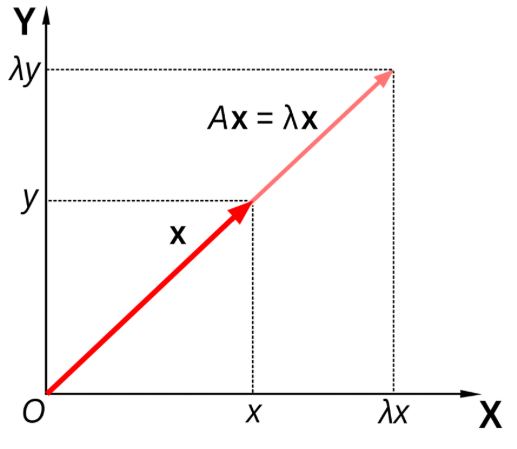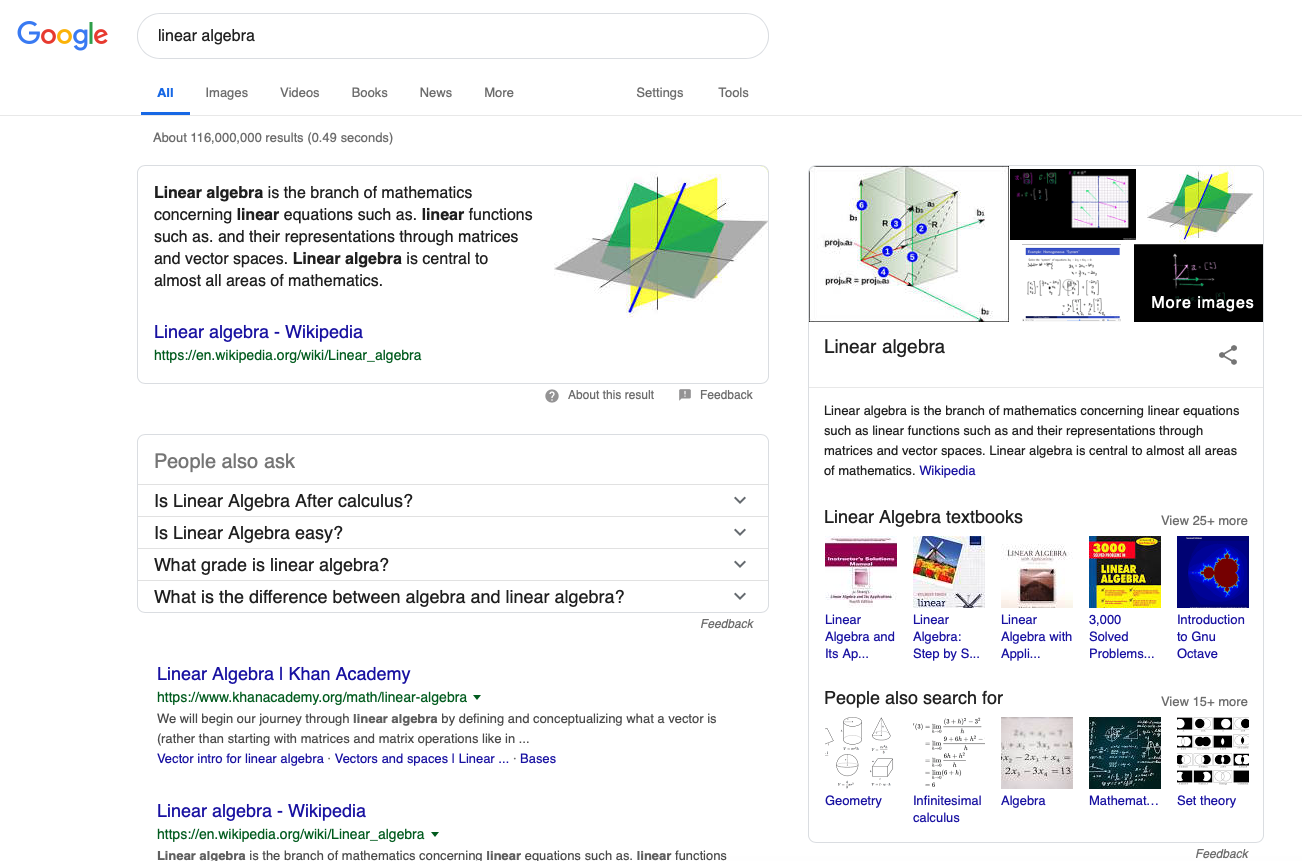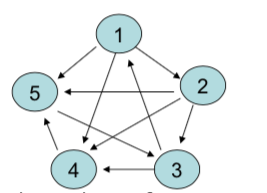School of Economics and Management
Beihang University
http://yanfei.site

## Why numerical linear algebra?

• Difference between linear algebra and applied linear algebra.
• Think about linear algebra in Statistics.
• In curve fitting.
• In image processing.
• In signal processing.
• etc.

## Eigenanalysis

• In general, a matrix acts on a vector by changing both its magnitude and its direction.
• However, a matrix may act on certain vectors by changing only their magnitude, and leaving their direction unchanged (or reversing it).
• these vectors are the eigenvectors of the matrix
• the scaling factor is the eigenvalue.## Example

• Think about the linear transformation $$\mathbf{y} = \mathbf{A}\mathbf{x}$$, where

$\mathbf{A} = \left(\begin{array}{cc} 2 & 0\\ 0 & 0 \end{array}\right),~\mathbf{x} = \left(\begin{array}{c} \cos\theta \\ \sin\theta \end{array}\right),~\mathbf{y} =\left(\begin{array}{c} y_1 \\ y_2 \end{array}\right).$

• What is its geometric interpretation?

• What happens when $$\theta = \frac{\pi}{4}$$?

• When $$\theta = \frac{\pi}{2}$$?

• When $$\theta = 0$$?

## Example

• What if $$\mathbf{A}$$ is not diagnal?
• How to find its eigenvalues and eigenvectors?
• If $$\mathbf{A}$$ is a real symmetric matrix
• Only real eigenvalues
• $$n$$ distinct linearly independent eigenvectors
• pairwise orthogonal
• $$\mathbf{A = Q\Lambda Q^T}$$
• When $$A$$ is diagonalisable?
• If a diagonalisation doesn't exist, there is always a triangularisation via Schur Decomposition: $$\mathbf{A = QSQ^T}$$.
• Let us revisit together the properties of eigenvalues and eigenvectors.

## What does eigenanalysis help to do?

• Let's try to understand what $$\mathbf{Ax} = \lambda \mathbf{x}$$ is really asking.
• Can we find a pair of $$\lambda$$ and $$\mathbf{x}$$ such that when a matrix $$\mathbf{A}$$ is applied to $$\mathbf{x}$$, it doesn't change the direction and just scales the vector?
• If we can find such a pair, then everytime we do something with $$\mathbf{Ax}$$ in some mathematical operation, we can replace it with $$\lambda \mathbf{x}$$.

## What does eigenanalysis help to do?

• Consider $$\mathbf{A} = \left(\begin{array}{cc} 5 & -1\\ -2 & 4 \end{array}\right)$$ and $$\mathbf{x} = \left(\begin{array}{c} 1 \\ -1 \end{array}\right)$$.
• what if we now want to calculate $$\mathbf{A^{20}x}$$?
• what if we now want to calculate $$\mathbf{A^{-1}x}$$?
• Computationally, we would rather work with scalars than matrices and this is what eigenanalysis helps us do.
• But what if we are not lucky enough to be asked to multiply a matrix by one of its eigenvectors?

## Advantages of eigenanalysis

• It enables us to replace a matrix with a scalar when we have the opportunity to change our coordinate system to the eigenvectors of a matrix.
• We can express any vector in terms of this new coordinate system.
• We can use the fact that $$\mathbf{Ax} = \lambda \mathbf{x}$$ to simplify calculations.

## Application of Eigenanalysis: Google## How to know page rank?

• How does the search engine know which pages are the most important?
• Google assigns a number to each individual page, expressing its importance.
• This number is known as the PageRank and is computed via the eigenvalue problem $$Aw = \lambda w$$, where $$A$$ is based on the link structure of the Internet.

• Suppose we have a set of webpages $$W$$, with $$|W|=n$$ as the number of webpages.
• We define a connectivity (adjacency) matrix $$A$$ as follows: $$A_{i,j}$$ is 1 if there is a hyperlink from page $$i$$ to page $$j$$ and 0 otherwise.
• Typically $$A$$ is huge (27.5 billion x 27.5 billion in 2011), but extremely sparse (lots of zero values)

## A small example

• Consider the small set of five webpages.• The connectivity matrix is $A = \left(\begin{array}{ccccc} 0 & 1 & 0 & 1 & 1\\ 0 & 0 & 1 & 1 & 1\\ 1 & 0 & 0 & 1 & 0\\ 0 & 0 & 0 & 0 & 1\\ 0 & 0 & 1 & 0 & 0 \end{array}\right)$

## A small example

• The ranking of page $$i$$ is proportional to the sum of the rankings of all pages that link to $$i$$: $$r_4 = \alpha(r_1 + r_2 + r_3)$$.
• So this is a system of $$n =5$$ linear equations: $r = \alpha A^T r, ~\text{or}~A^T r = (1/\alpha) r.$
• The ranking vector is treated like a probability of relevance, so we need to then rescale so that $$\Sigma_{i=1}^n = 1.$$
• Go to R and compute pageranks.

## What you need to realise for now

• Finding the eigenvalues and eigenvectors of a massive matrix is computationally challenging though (don’t try to solve the characteristic polynomial!)
• and you will learn numerical techniques later.